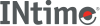﻿ divINtime SDK Help
div

Divides the numerator by the denominator, computing the quotient and the remainder of two integer values.

```#include <stdlib.h>

div_t div (int numer, int denom);
```

#### Parameters

`numer`
Numerator.
`denom`
Denominator. If 0, errno is set to EDOM and 0 returned for quotient and remainder.

#### Return Values

A div_t structure, described in STDLIB.H.

The sign of the quotient is the same as that of the mathematical quotient. Its absolute value is the largest integer that is less than the absolute value of the mathematical quotient.

#### Requirements

Versions Defined in Include Link to
INtime 3.0 intime/rt/include/stdlib.h stdlib.h clib.lib